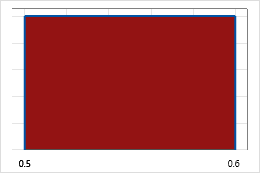# Uniform distribution

Use the uniform distribution to describe continuous variables that have a constant probability. For example, a population of parts range from 0.5 to 0.6 cm long. If every value between 0.5 and 0.6 cm has an equal likelihood of occurring, these data follow a uniform distribution.

The uniform distribution is a continuous distribution that models a range of equally likely values. The uniform distribution is specified by lower and upper end points. For example, the following graph illustrates a uniform distribution.The uniform distribution does not occur often in nature, but it is important as a reference distribution. The uniform distribution is also known as the rectangular distribution.

By using this site you agree to the use of cookies for analytics and personalized content.  Read our policy